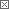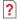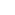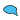Previous in Forum: Hydrogen Sulphide and Steam Reaction? Next in Forum: How Does a Gas Sniffer Work?Power-User

Join Date: Apr 2007
Location: Austin, TX
Posts: 368

#Discharge Coefficient in Choked Flow

02/24/2009 10:32 AM

Hello, I am trying to calculate the choked flow through a globe valve in order to determine a max flow case. The flow is definitely sonic and I found an equation online that will calculate the choked flow in lbm/s:

w = Cd * A * P * SQRT(g*k*M/(Z*R*T))*(2/(k+1))^((k+1)/(2k-2))

at this website:

http://www.air-dispersion.com/usource.html

I found a units error where they are using the gravitational constant instead of the correction factor, but that doesn't change the result since they are both 32.2.

Perry's eqn. 6.122 is similar albeit a little less worked out:

G = P * SQRT((2/(k+1))^((k+1)/(k-1))*k*g*M/(R*T))

G is the mass flux rate and then I can multiply by the orifice area to get the flow rate in lbm/s.

The big difference between the equations is the C term in the first equation, the coefficient of discharge which I would expect to see in a flow calculation. The Perry's equation assumes a frictionless nozzle and obviously that is a squirrely assumption but is the C term in the first equation there to account for frictional losses?

__________________
Money doesn't talk, it screams in your face.
Pathfinder Tags: Discharge coefficient flow valve
Interested in this topic? By joining CR4 you can "subscribe" to
Anonymous Poster#1

###Re: Discharge Coefficient in Choked Flow

02/25/2009 8:38 AM

Two things going on with the equations. The first has Cd, "flow discharge coefficient", and Z , "compressibility factor". Cd is a factor to account for vena contracta effects, and is generally on the order of 0.6 [ sharp edged orifice ] to 0.8 [ longer hole ]. Z is a factor to account for differences between the compressiblity of a real compressible gas and an ideal gas.

The second equation, having neither Cd or Z, is for ideal compressible flow. The first equation is the one you need. If your gas is ideal (i.e. air at room temp or higher), then Z = 1. For the Cd, specific data on the globe valve is needed. If possible, contact the manufacturer to see if any compressible Cd curves, or loss curves, are available for the valve. If not, estimate the minimum flow area in the valve's position, and band the possible flow range using 0.6 < Cd < 1.0.

Power-User

Join Date: Nov 2006
Location: Oregon
Posts: 241#2

###Re: Discharge Coefficient in Choked Flow

02/25/2009 4:31 PM

Nobody gives too much info anymore. Sounds like a song title. If you are using a gas other than compressed air modify the results of this by the density of the gas with air being one (1).

Q scfm = 14.5 x D"2 x (P1 x 14.7)psia if you know the diameter and don't choke the flow with small fittings and tubing with less flow diameter than the valve.

If you don't know the diameter or don't trust the plumbing this is quick and deadly accurate.

1. Fill a tank to the pressure you are interested in.
2. Connect the inlet of the valve to the tank with outlet open to atmosphere.
3. Simultaneously open the valve and start a timer.
4. Stop the timer when the pressure has fallen to 37% (.3678) of P1 psig.*
5. The time you noted is a time constant, Tk
6. V tank volume ft3 x P1 psig* x 60 / (14.7 x Tk) = Q scfm

* In the equation for Q use (P1 + 14.7). In the flow test use gauge pressure.

For the flow test the tank cannot decay below 1 atmosphere. The differential from pressurized to depressurized is the difference in gauge pressure.